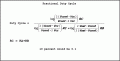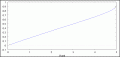# 555 control voltage used for modulating output voltage in astable mode

#### denison

Joined Oct 13, 2018
312
does anybody know a formula for calculating the duty cycle of the 555 using the control voltage terminal? If this terminal is not used the formula is well known when 2 resistor values and a capacitor value is known.
I need a formula which also includes the voltage at the CV terminal.

#### AnalogKid

Joined Aug 1, 2013
10,176
The relationship of the CV to the output frequency varies among 555 types. Both the bipolar and CMOS 555s are based on a string of three identical resistors, and one of the string nodes is brought out as the CV input. Once you know the internal resistor value and the externally-applied control voltage, you can calculate the shift in duty cycle and frequency with variations of Ohm's Law.

ak

#### denison

Joined Oct 13, 2018
312
The relationship of the CV to the output frequency varies among 555 types. Both the bipolar and CMOS 555s are based on a string of three identical resistors, and one of the string nodes is brought out as the CV input. Once you know the internal resistor value and the externally-applied control voltage, you can calculate the shift in duty cycle and frequency with variations of Ohm's Law.

ak

#### denison

Joined Oct 13, 2018
312
hi Analog Kid, I am very familiar with the 555. Lets just consider the bipolar 555. I never touch cmos types because they are too easily damaged by static electricity. I have the ne555.
I need the equation which includes the 2 resistors and capacitor and the CV input voltage. If I put in the values of these I need the equation which will give me the duty cycle and period. I am talking about the resistors and capacitor you add to your circuit, not the internal resistors of the 555.
My text book does not give me the equation for the duty cycle if there is a voltage input at the CV terminal.
A 555 data sheet may have this info but I doubt it. If I knew how the equation was derived I may be able to determine the equation with a voltage at the CV terminal.

#### bertus

Joined Apr 5, 2008
22,120
Hello,

Have a look at page 13 of the attached datasheet.

Bertus

#### Attachments

• 569.8 KB Views: 47

#### denison

Joined Oct 13, 2018
312
Hello,

Have a look at page 13 of the attached datasheet.

Bertus
My modulation input is a fixed voltage which I will change as necessary to get the duty cycle I require. I need an equation for this. The equation will include 2 resistor values, a capacitor value and a voltage at the CV input. Most sources only include the equation for the first 2.
Hello,.

Have a look at page 13 of the attached datasheet.

Bertus

#### MrAl

Joined Jun 17, 2014
9,633
My modulation input is a fixed voltage which I will change as necessary to get the duty cycle I require. I need an equation for this. The equation will include 2 resistor values, a capacitor value and a voltage at the CV input. Most sources only include the equation for the first 2.

This i had derived some time ago might be on this forum. If not we can derive it once more. Then internals are given in the data sheet

#### Kjeldgaard

Joined Apr 7, 2016
475
I don't think there is enough information on the table yet.

It is limited how much Duty Cycle can be changed with the help of the Control Input. At some point you reach beyond the Common Mode limits of the two comparators, and I do not recall having seen these values in any data sheet.

#### MrAl

Joined Jun 17, 2014
9,633
I don't think there is enough information on the table yet.

It is limited how much Duty Cycle can be changed with the help of the Control Input. At some point you reach beyond the Common Mode limits of the two comparators, and I do not recall having seen these values in any data sheet.
It should not be hard to find out. The main thing is that we have a formula to start with, then experiment to find out how much we can take it.
What the limits are.

Joined Mar 10, 2018
4,057
Are you looking for a duty cycle only V control, one that does not also
vary frequency ?

Curious, no specs for freq accuracy or range, duty cycle accuracy or range....

Regards, Dana.

#### Alec_t

Joined Sep 17, 2013
13,229
The equation will include 2 resistor values, a capacitor value and a voltage at the CV input.
Methinks the equation will also involve the supply voltage Vcc, because (a) that affects the cap charge rate, and (b) the upper input threshold voltage Vthr and lower threshold Vtrig will no longer each be a fixed fraction of Vcc. Without the control voltage, Vthr = 2Vcc/3 and Vtrig = Vcc/3; but with a low source impedance control voltage Vcon, Vthr = Vcon and Vtrig = Vcon/2.

#### AnalogKid

Joined Aug 1, 2013
10,176
A 555 data sheet may have this info but I doubt it.
Might I suggest that you replace that doubt with certainty?

There are two modulation circuits on the datasheet. Which one fits your requirements?

ak

#### eetech00

Joined Jun 8, 2013
3,418
Hello

There is a PWM circuit in app note AN-170 that uses the control pin with op amp.

Figure 22 Voltage to Pulse Duration Converter.

eT

#### djsfantasi

Joined Apr 11, 2010
8,672
<wrong link> on using a 555 for a pulse width modulation circuit includes an equation for the component values. I just searched for it and this onecame up. My search criteria was “555 pulse width modulation calculator”.

Note: posted the wrong link in the original post

Last edited:

#### AnalogKid

Joined Aug 1, 2013
10,176
This article on using a 555 for a pulse width modulation circuit includes an equation for the component values. I just searched for it and this one came up. My search criteria was “555 pulse width modulation calculator”.
Those articles are for a manually-adjusted pulse width oscillator, not one for electric modulation.

ak

#### djsfantasi

Joined Apr 11, 2010
8,672
Those articles are for a manually-adjusted pulse width oscillator, not one for electric modulation.

ak
Yes, it is.

I pasted the wrong link and now (of course) I’m having trouble finding the one I meant.

@denison , sorry for the confusion!

This doesn’t include the equation, but should be an example of the circuit you need.

#### MrAl

Joined Jun 17, 2014
9,633
Hello again,

Here is an expression for the fractional duty cycle in astable operation.
This means that 0.1 means 10 percent duty cycle.

DC=(log((2*Vsat-Vcont)/(2*(Vsat-Vcont)))*RB)/(log((2*(Vcont-Vcc))/(Vcont-2*Vcc))*RC+log((2*Vsat-Vcont)/(2*(Vsat-Vcont)))*RB)

where:
log() is the natural log ln(),
Vsat is the saturation voltage of the discharge transistor,
Vcont is the control voltage,
RB is the lower resistor connected to the capacitor,
RA is the upper resistor connected to Vcc,
RC is RC=RA+RB,
Vcc is the supply voltage (positive),
C is the capacitor but it does not have to appear in the formula for duty cycle.

When the control voltage is varied between 5v and 0v the duty cycle varies from around 0 to 100 percent. However, the limits of the input of the comparator are not known so it may only go up to maybe 4v and down to maybe 0.5v for proper operation. This should be tested with a breadboard.Last edited:
•Alec_t

#### Hymie

Joined Mar 30, 2018
1,102
My 555 timer cookbook advises that the mathematics relating to the relationship between frequency and control voltage is complex due to the exponential charging of the capacitor.

However if a constant current source is used to charge the RC timing components then the relationship between charging time and control voltage will be relatively linear.

#### MrAl

Joined Jun 17, 2014
9,633
My 555 timer cookbook advises that the mathematics relating to the relationship between frequency and control voltage is complex due to the exponential charging of the capacitor.

However if a constant current source is used to charge the RC timing components then the relationship between charging time and control voltage will be relatively linear.
Hi,

Not that complex see post before yours.